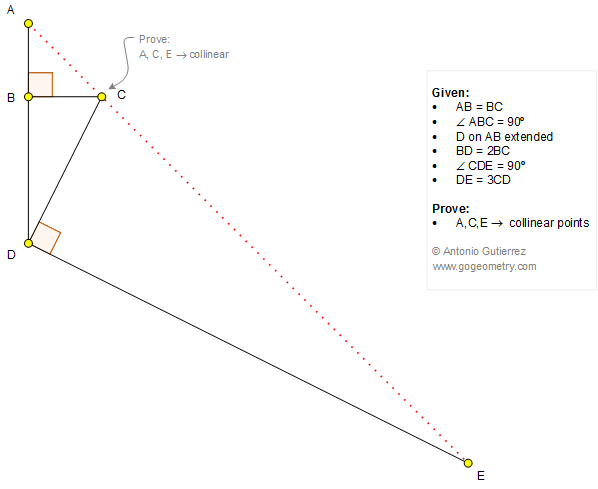# Geometry Problem 1255

### Elements: Right Triangle, Angle, 90 Degrees, Perpendicular, Collinear Points

In the figure below, AB = BC and the angle ABC = 90 degrees. A point D is on AB extended so that BD = 2BC. The angle CDE = 90 degrees so that DE = 3CD. Prove that the points A, C, and E are collinear.